Open In App

# SQL Query to Add Email Validation Using Only One Query

In this article let us see how can we check for the validation of mails in the student database using MSSQL as the database server. For example, if the email is like abcdedfABCDEF…0123456@gmail.com this is the valid form of an email if it is other than this then that is said to Invalid. So, now we will discuss this concept in detail step-by-step:

Step 1: Creating a database college by using the following SQL query as follows.

`CREATE DATABASE college;`

Step 2: Using the database student using the following SQL query as follows.

`USE college;`

Step 3: Creating a table student with 4 columns using the following SQL query as follows.

```CREATE TABLE student
(
s_id varchar(20),
s_name varchar(20),
s_branch varchar(20),
s_email varchar(100)
);```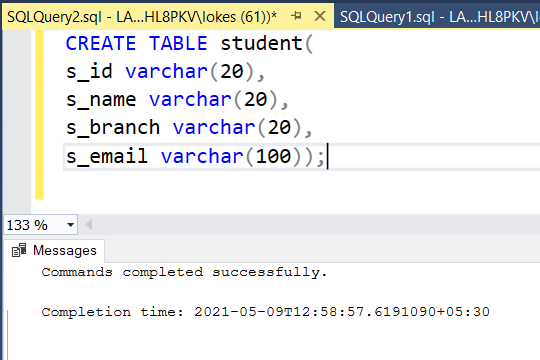Step 4: To view the description of the table in the database using the following SQL query as follows.

`EXEC sp_columns student;`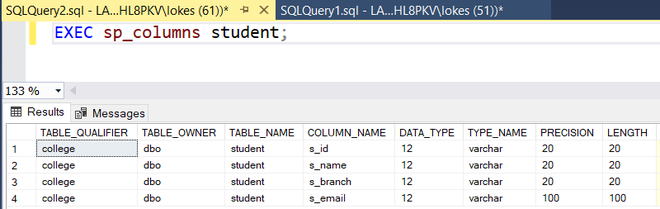Step 5: Inserting rows into student_details table using the following SQL query as follows.

```INSERT INTO student VALUES
('19102001','JOHNSON','E.C.E','john432@gmail.com'),
('19102002','VIVEK','E.C.E','VIVEK2252gmail.com'),
('19102003','DINESH','E.C.E','dineshg@gmail.com'),
('19102004','HARSHA','E.C.E','hARsha302@gmail.com'),
('19102005','DAVID','E.C.E','david2000@gmail.com'),
('19102006','NAVIN','E.C.E','navin00'),
('19102007','VINAY','E.C.E','Vinay24mail.com');```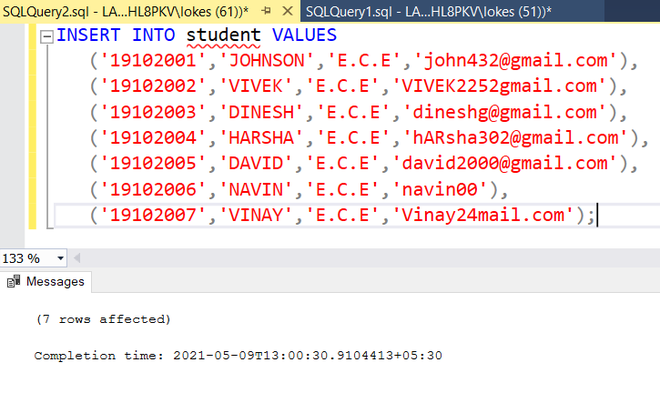Step 6: Viewing the table student_details after inserting rows by using the following SQL query as follows.

`SELECT * FROM student;`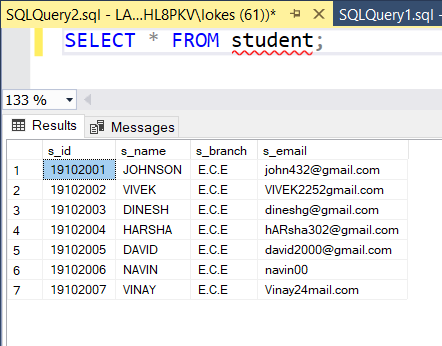Query: Now we are querying to display all the student details with valid emails. So, the email validation is done by using pattern matching which can be done by using LIKE operator in MSSQL. It checks for the fixed pattern set by the database designer in the data and displays the data that matches the fixed pattern.

Syntax:

```SELECT * FROM table_name
WHERE attribute LIKE 'pattern';```
```SELECT * FROM student
WHERE s_email LIKE '%@gmail.com';```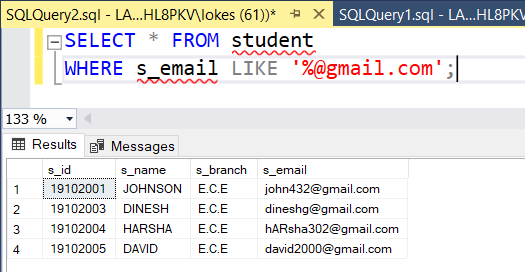Only the students with valid emails are displayed in the tables.